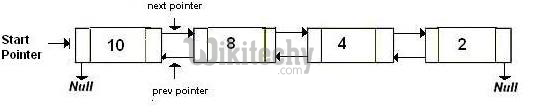# QuickSort on Doubly Linked List

Quick Sort on Doubly Linked List - Doubly Linked List - The partition function for linked list is also similar to partition for arrays.

Java Program: QuickSort on doubly linked list

Following is a typical recursive implementation of QuickSort for arrays. The implementation uses last element as pivot.

``````/* A typical recursive implementation of Quicksort for array*/

/* This function takes last element as pivot, places the pivot element at its
correct position in sorted array, and places all smaller (smaller than
pivot) to left of pivot and all greater elements to right of pivot */
int partition (int arr[], int l, int h)
{
int x = arr[h];
int i = (l - 1);

for (int j = l; j <= h- 1; j++)
{
if (arr[j] <= x)
{
i++;
swap (&arr[i], &arr[j]);
}
}
swap (&arr[i + 1], &arr[h]);
return (i + 1);
}

/* A[] --> Array to be sorted, l  --> Starting index, h  --> Ending index */
void quickSort(int A[], int l, int h)
{
if (l < h)
{
int p = partition(A, l, h); /* Partitioning index */
quickSort(A, l, p - 1);
quickSort(A, p + 1, h);
}
}``````

### Can we use same algorithm for Linked List?

Following is Java implementation for doubly linked list. The idea is simple, we first find out pointer to last node. Once we have pointer to last node, we can recursively sort the linked list using pointers to first and last nodes of linked list, similar to the above recursive function where we pass indexes of first and last array elements. The partition function for linked list is also similar to partition for arrays. Instead of returning index of the pivot element, it returns pointer to the pivot element. In the following implementation, quickSort() is just a wrapper function, the main recursive function is _quickSort() which is similar to quickSort() for array implementation.

READ  How To Install Cassandra on CentOS 6

###Java Programming for QuickSort on Doubly Linked List

``````// A Java program to sort a linked list using Quicksort

/* a node of the doubly linked list */
static class Node{
private int data;
private Node next;
private Node prev;

Node(int d){
data = d;
next = null;
prev = null;
}
}

// A utility function to find last node of linked list
Node lastNode(Node node){
while(node.next!=null)
node = node.next;
return node;
}

/* Considers last element as pivot, places the pivot element at its
correct position in sorted array, and places all smaller (smaller than
pivot) to left of pivot and all greater elements to right of pivot */
Node partition(Node l,Node h)
{
// set pivot as h element
int x = h.data;

// similar to i = l-1 for array implementation
Node i = l.prev;

// Similar to "for (int j = l; j <= h- 1; j++)"
for(Node j=l; j!=h; j=j.next)
{
if(j.data <= x)
{
// Similar to i++ for array
i = (i==null) ? l : i.next;
int temp = i.data;
i.data = j.data;
j.data = temp;
}
}
i = (i==null) ? l : i.next;  // Similar to i++
int temp = i.data;
i.data = h.data;
h.data = temp;
return i;
}

/* A recursive implementation of quicksort for linked list */
void _quickSort(Node l,Node h)
{
if(h!=null && l!=h && l!=h.next){
Node temp = partition(l,h);
_quickSort(l,temp.prev);
_quickSort(temp.next,h);
}
}

// The main function to sort a linked list. It mainly calls _quickSort()
public void quickSort(Node node)
{
// Find last node

// Call the recursive QuickSort
}

// A utility function to print contents of arr
{
}
}

/* Function to insert a node at the beginging of the Doubly Linked List */
void push(int new_Data)
{
Node new_Node = new Node(new_Data);     /* allocate node */

return;
}

/* link the old list off the new node */

/* change prev of head node to new node */

/* since we are adding at the begining, prev is always NULL */
new_Node.prev = null;

/* move the head to point to the new node */
}

/* Driver program to test above function */
public static void main(String[] args){

list.push(5);
list.push(20);
list.push(4);
list.push(3);
list.push(30);

}
}``````

### Output :

```Linked List before sorting
30  3  4  20  5
3  4  5  20  30```

Time Complexity: Time complexity of the above implementation is same as time complexity of QuickSort() for arrays. It takes O(n^2) time in worst case and O(nLogn) in average and best cases. The worst case occurs when the linked list is already sorted.

READ  JAVA Programming - Find common elements in three sorted arrays

### Can we implement random quick sort for linked list?

Quicksort can be implemented for Linked List only when we can pick a fixed point as pivot (like last element in above implementation). Random QuickSort cannot be efficiently implemented for Linked Lists by picking random pivot.#### Venkatesan Prabu

Wikitechy Founder, Author, International Speaker, and Job Consultant. My role as the CEO of Wikitechy, I help businesses build their next generation digital platforms and help with their product innovation and growth strategy. I'm a frequent speaker at tech conferences and events.

X